# Extract Date from a Date and Time

This post will guide you how to extract date part from a date with time values in Excel. How do I remove time part from a date in Excel. How to get only date part from the date with time values via Format cells feature or Excel function. How to extract date from a date and time with VBA code in Excel.

## Extract date with Format Cells

You can use the Format cells feature to extract date from a date and time value, just do the following steps:

#1 select the cells from which you want to extract date

#2 right click on those cells, select Format Cells… menu from the drop down menu list. And the Format Cells window will appear.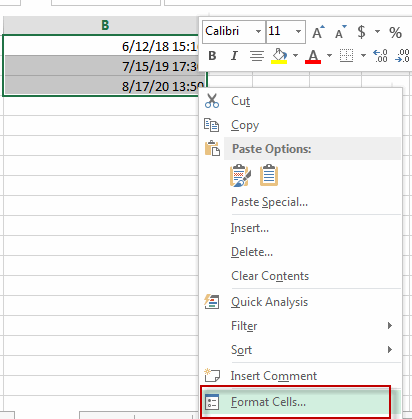#3 switch to Number tab, choose Date category under Category: section. Then select one type of dates such as: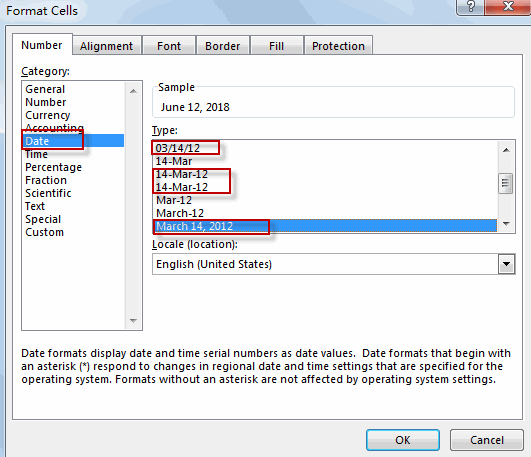#4 click OK button, you will see that the date has been extracted from each date and time value.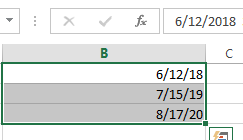## Extract date with Function

The date is represented as serial number and times are fractional values in Excel. So if you want to only display the date part, just using the INT function to remove time portion. Or you can also use the TRUNC function to discard the time portion.

Type the below formula in formula box:

=INT(B1)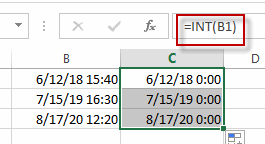Or

=TRUNC(B1)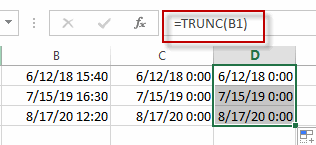Note: you should make sure the date with time values in one cell is a date format.

Extract date with VBA Code

You can also write an Excel VBA Marco to extract date portion from a date with time values in Excel. Just do the following steps:

1# click on “Visual Basic” command under DEVELOPER Tab.2# then the “Visual Basic Editor” window will appear.

3# click “Insert” ->”Module” to create a new module.4# paste the below VBA code into the code window. Then clicking “Save” button.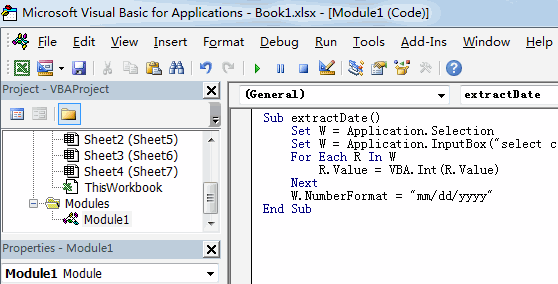```Sub extractDate()
Set W = Application.Selection
Set W = Application.InputBox("select cells of Range:", "extract date from a date and time", W.Address, Type:=8)
For Each R In W
R.Value = VBA.Int(R.Value)
Next
W.NumberFormat = "mm/dd/yyyy"
End Sub```

5# back to the current worksheet, then run the above excel macro. Click Run button.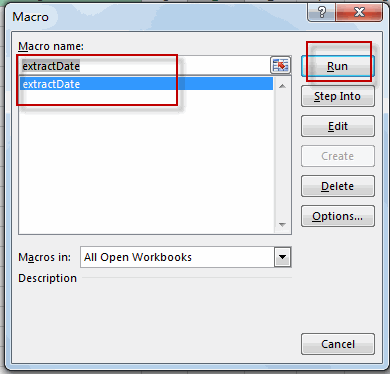6# Select one range from which you want to extract date portion.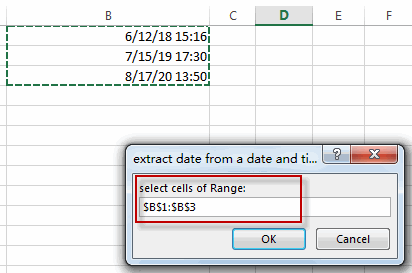#7 Let’s see the result: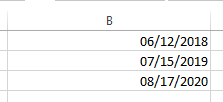### Related Functions

• Excel INT function
The Excel INT function returns the integer portion of a given number. And it will rounds a given number down to the nearest integer.The syntax of the INT function is as below:= INT (number)…
• Excel TRUNC function
The Excel TRUNC function truncates a number to an integer by removing the fractional part of the number.The syntax of the TRUNC function is as below:=TRUNC (number, number_digits)…
Related Posts

Find and Replace Multiple Values

This post will guide you how to find and replace multiple values at once with VBA macro or using formula  in Excel. How do I make multiple find and replace in Excel. Suppose that you have a few cells containing ...

VBA Macro For VLOOKUP From Another Sheet

In the previous post, you should know that how to fix or remove the #N/A error when using VLOOKUP formula to lookup value from another sheet. And this post will show you how to use VBA code to vlookup data ...

How To Insert Comments in Protected Worksheet in Excel

This post will show you how to allow comments in a protected worksheet in Excel. You can easily to insert comments into cells in a normal worksheet in Excel, but if want to insert a comment in a worksheet that ...

How To Convert Text to Upper Cases(Using VBA) in Excel

This post will show you how to switch from lower case to upper case in Excel. and I am going to show you two different ways of converting text to upper cases using formula or VBA macro in Excel 2013,Excel ...

How To Hide Every Other Row in Excel (Using VBA)

This post will show you how to hide alternate rows or columns in Excel or how to hide every third, fourth, fifth row or column in Excel. If you want to hide every other row in your current worksheet, how ...

How to Disable the Save As Prompt in Excel

This post will show you how to use a VBA Macro to save an Excel file and overwrite any existing file without a prompt so that you are going to get the little window that says file already exists do ...

How to Count Cells that Contain even or odd numbers in Excel

This post will guide you how to count the number of cells that contain odd or even numbers within a range of cells using a formula in Excel 2013/2016.How do I count cells that contain odd numbers through the use ...

How to Count Cells that Contain negative Numbers in Excel

This post will guide you how to count the number of cells that contain negative numbers within a range of cells using a formula in Excel 2013/2016.You can count the number of negative numbers in your data using easy functions ...

How to Count Cells Are Not Blank or Empty in Excel

This post will guide you how to count cells that are not blank or empty in a given range cells using a formula in Excel 2013/2016.How do I count the number of cells that are not blank in a particular ...

How to Count Cells Less Than a Specific Value in Excel

This post will guide you how to count the number of cells less than a particular numeric value in a given range cells using a formula in Excel 2013/2016. How do I count cells that are less than a specific ...

Sidebar